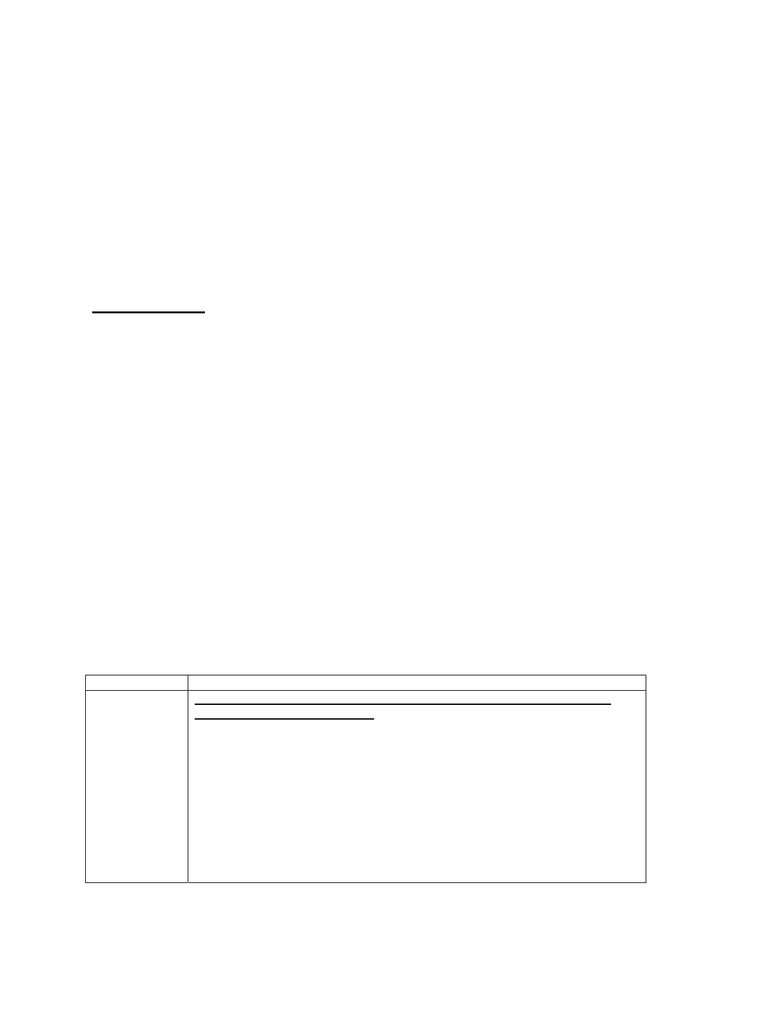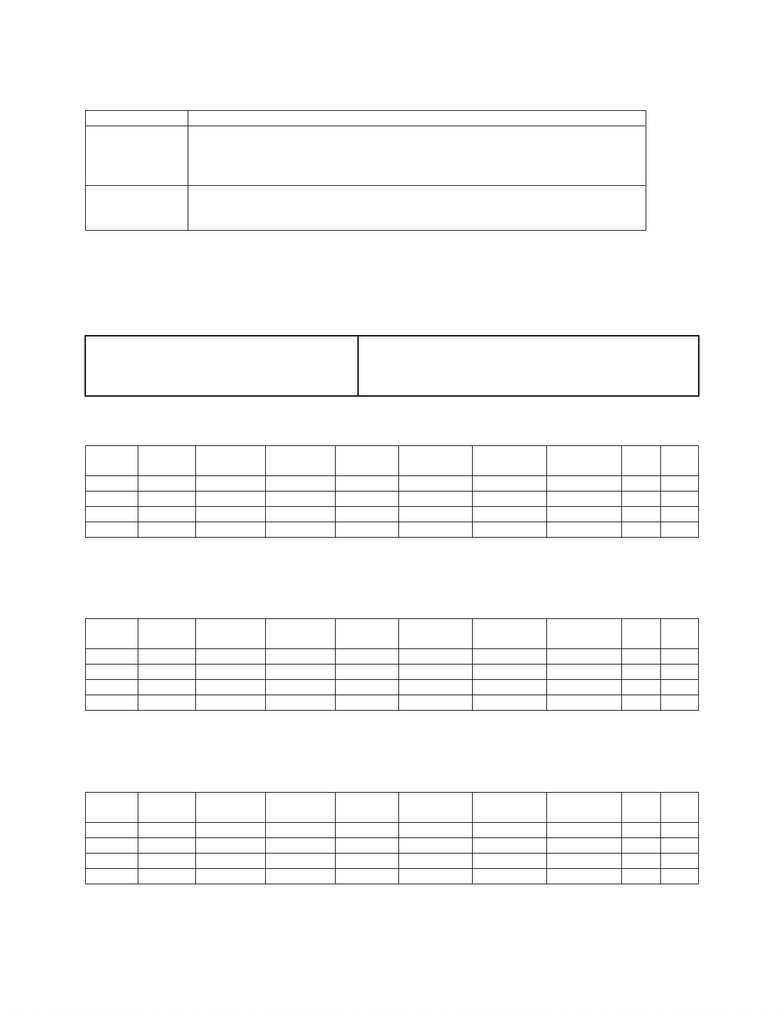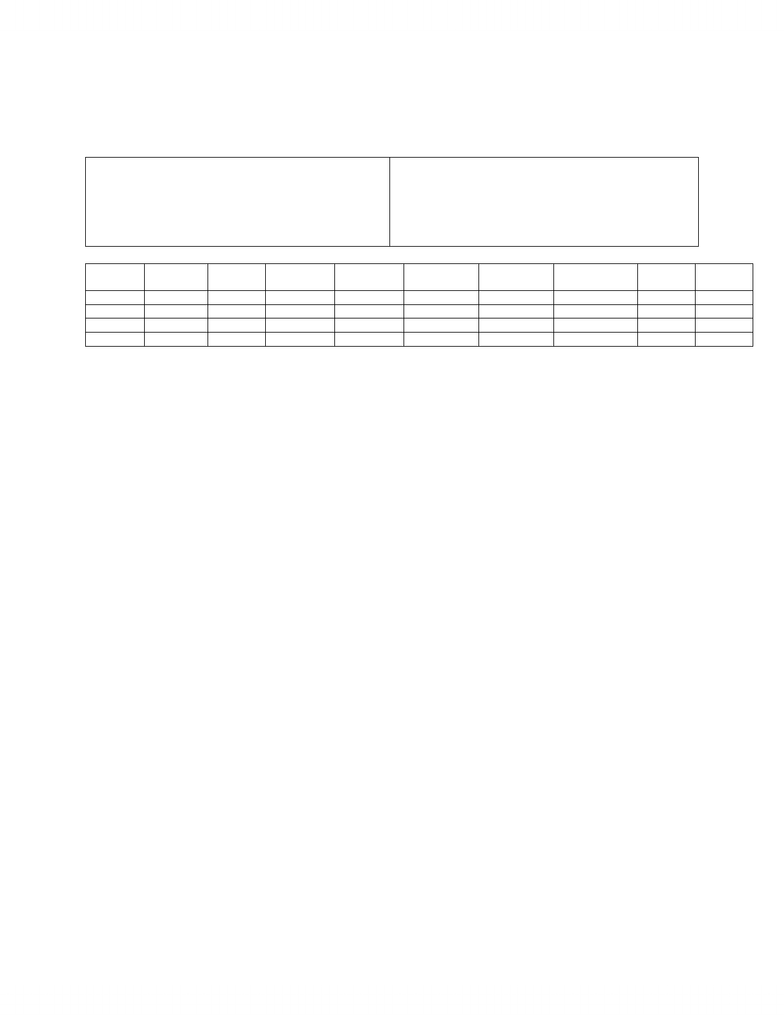Study Guides (400,000)
CA (160,000)
Western (10,000)
MOS (1,000)
Midterm

# Management and Organizational Studies 3330A/B Study Guide - Midterm Guide: 5,6,7,8, Indian Railways, Manufacturing Resource Planning

Department
Management and Organizational Studies
Course Code
MOS 3330A/B
Professor
Rick Burjaw
Study Guide
Midterm

This preview shows pages 1-3. to view the full 12 pages of the document.Test 2 Review Problems and Solutions
A. Computational Questions
(i) Review computational examples in the lecture notes.
(ii) Study questions from the old exams – more straightforward than the textbook
questions.
(iii) Study end-of-chapter problems in the textbook.
Note on rounding:
General rule #1: In most cases, just use “normal” rounding. For example, 32.15 should be
rounded down to 32 if the final answer has to be an integer (e.g., order quantity). This
works for EOQ, EPQ and forecasting.
General rule #2: For all intermediate calculations, use the decimal numbers (2 or 3
decimal places will suffice). For example, one question asks you to calculate EOQ and
the total cost. Let’s say that EOQ = 32.15. Then 32.15 will be rounded down to 32 in
your final statement of what the order quantity is. In order to calculate the total cost,
however, use 32.15 in the TC formula.
Exception: For example, EOQ = 32.15 and rounded down to 32. Let’s say that the annual
demand is 1000. Based on these numbers, the number of orders = D/Q = 1000/32.15 =
31.1. Now, in the final statement, the optimal order quantity is 32, and if the number of
orders is 31, then the total demand satisfied = 31×32 = 992, which is less than 1000. Only
in this kind of situation, the number of orders may be rounded up to 32 from 31.1, and
state in the final answer that 32 orders are necessary to satisfy all of demand based on
EOQ = 32.
A1. Textbook Computational Problems
Topic Textbook End-of-Chapter Problems
Aggregate
Planning USE THE SAME TABLE FORMAT AND COLUMN HEADINGS AS THE
LECTURE NOTE EXAMPLES.
#1
#2 – textbook refers to it as level but it really is hybrid
#3
#4
#5 – textbook refers to it as chase but it really is hybrid
#6 – solution different from the textbook is provided
#7 – solution different from the textbook is provided
#9 – textbook refers to it as level but it really is hybrid
#11 – textbook refers to it as chase but it really is hybrid
#13
1

Only pages 1-3 are available for preview. Some parts have been intentionally blurred.Topic Textbook End-of-Chapter Problems
Resource
Planning (MRP) #1
#2
#3
#4
Scheduling #5
#7
#8
A2. Computational Questions from Old Exams
1. University Press publishes textbooks for the academic market. The relevant cost and printing
equipment-related capacity information are given below.
Regular production cost = \$20 per book Regular production capacity = 10 000 books per quarter
Overtime production cost = \$30 per book Overtime production capacity = 5000 books per quarter
Subcontracting cost = \$35 per book Subcontracting capacity = unlimited
Holding cost = \$2 per book Beginning inventory = 0
a) Fill all blank spaces in the following table so that it represents an initial aggregate production plan
using a pure level approach with average demand. What is the total cost of the initial plan?
Quarter Demand Total
Production Workforce Inventory Regular
Production Overtime
Production Subcontract Hire Fire
1 5000
2 10000
3 30000
4 25000
b) Fill all blank spaces in the following table so that it represents an initial aggregate production plan
using a pure chase approach. For this question, we will ignore workforce-related costs. What is
the total cost of the initial plan? Compared to the level approach in part a), which approach seems
Quarter Demand Total
Production Workforce Inventory Regular
Production Overtime
Production Subcontract Hire Fire
1 5000
2 10000
3 30000
4 25000
c) Based on parts a) and b), develop an improved aggregate production plan in terms of the total
cost. The plan may deviate from a pure level or a pure chase approach. Assume that no other
information or objective will be considered. Provide the total cost of the improved plan and
explain briefly how you came up with your improved plan.
Quarter Demand Total
Production Workforce Inventory Regular
Production Overtime
Production Subcontract Hire Fire
1 5000
2 10000
3 30000
4 25000
2

Only pages 1-3 are available for preview. Some parts have been intentionally blurred.2. Wetski currently maintains a steady production level at the average demand all year around and does
not hire or fire workers. Fill all blank spaces in the following table so that it represents an aggregate
production plan based on Wetski’s current production and hiring policy. What is the total cost of this
plan?
Regular production cost = \$55 per unit Production rate = 750 units/worker/quarter
Overtime production cost = \$82 per unit Holding cost = \$4 per unit per quarter
Subcontracting cost = \$77 per unit Backorder cost = \$80 per unit per quarter
Opening workforce level = 11 Hiring cost = \$140 per worker
Overtime capacity = 2500 units per quarter Firing cost = \$550 per worker
Subcontracting capacity = 1000 units per quarter Opening inventory = 0
Quarter Demand
(units) Total
Prod. Workforce Inventory Regular
Prod. Overtime
Prod. Subcontract Hiring Firing
Fall 3700
Winter 4600
Spring 14500
Summer 21000
3